xIntensity (heat transfer)Encyclopedia
In the field of heat transfer
Heat transfer
Heat transfer is a discipline of thermal engineering that concerns the exchange of thermal energy from one physical system to another. Heat transfer is classified into various mechanisms, such as heat conduction, convection, thermal radiation, and phase-change transfer...

, intensity of radiation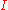is a measure of the distribution of radiant heat flux per unit area and solid angle
Solid angle
The solid angle, Ω, is the two-dimensional angle in three-dimensional space that an object subtends at a point. It is a measure of how large that object appears to an observer looking from that point...

, in a particular direction, defined according to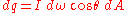where
•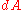is the infinitesimal source area
•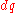is the outgoing heat transfer from the area•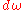is the solid angle
Solid angle
The solid angle, Ω, is the two-dimensional angle in three-dimensional space that an object subtends at a point. It is a measure of how large that object appears to an observer looking from that point...

subtended by the infinitesimal 'target' (or 'aperture') area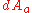•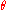is the angle between the source area normal vector and the line-of-sight between the source and the target areas.

Typical units of intensity are W·m-2·sr-1.

Intensity can sometimes be called radiance
Radiance and spectral radiance are radiometric measures that describe the amount of radiation such as light or radiant heat that passes through or is emitted from a particular area, and falls within a given solid angle in a specified direction. They are used to characterize both emission from...

, especially in other fields of study.

The emissive power of a surface can be determined by integrating the intensity of emitted radiation over a hemisphere surrounding the surface: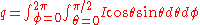For diffuse emitters, the emitted radiation intensity is the same in all directions, with the result that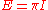The factor(which really should have the units of steradian
The steradian is the SI unit of solid angle. It is used to describe two-dimensional angular spans in three-dimensional space, analogous to the way in which the radian describes angles in a plane...

s) is a result of the fact that intensity is defined to exclude the effect of reduced view factor at large values; note that the solid angle corresponding to a hemisphere is equal to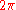steradians.

Spectral intensity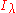is the corresponding spectral measurement of intensity; in other words, the intensity as a function of wavelength